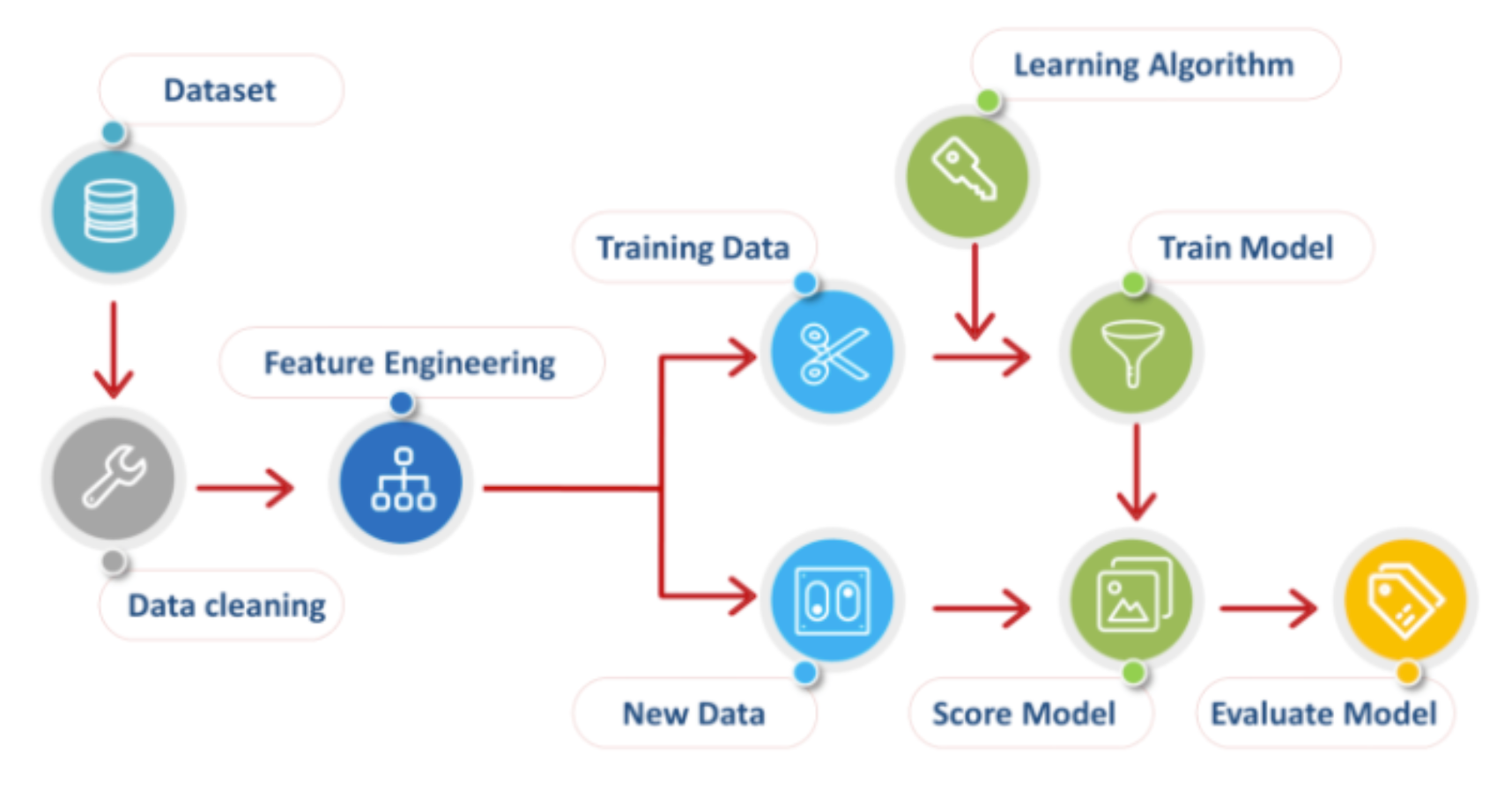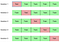# Cross Validation and Classification Metrics

The fastest and most simple way to evaluate a model is to perform train-test-split. This procedure, as its name suggests, splits the data into a training and testing set, trains the model using the training set data and checks the accuracy. However, can you rely on this alone when finalizing your model?

The simplest answer is no because of something called the accuracy paradox.

Let’s dive into the process for evaluating your machine learning model and using the best, most effective metrics to do so.Photo by Afroz Chakure

# Model Evaluation

Right off the bat, we take our data and begin the exciting (read laborious) process of cleaning and feature engineering. Once we are happy with the state of our data set, we can split all of the data into a training set and into a “new data” set that we use to test our model with.

The typical ratio for splitting the data set into train and test is 80:20, however, this is up to the Data Scientist’s discretion and can be tweaked as needed.

In the above code, the original data set of features is X and y is the corresponding target variables. In this example, we split the data 80:20, as noted by the ‘test_size = 0.2’ parameter.

As we were originally discussing, checking the model performance using only the accuracy metric for the test set is not adequate. For this reason, we need stronger, more effective evaluation metrics.

# Confusion Matrix

Enter the confusion matrix. In order to better understand this metric, let’s work with Sci-kit Learn’s breast_cancer data set. In this data set, malignant tumors are labelled as 1and benign tumors are labelled as 0.

For this example, we have already prepared our model using the .fit() method and calculated the predictions using the .predict(). We passed our ‘y_test’ and ‘predictions’ arrays into Sci-kit Learn’s confusion_matrix() and transformed this matrix to a DataFrame to look something like this:

Where we predicted the following instances:

1. True Positive: 90
2. False Negative: 0
3. False Positive: 6
4. True Negative: 47

There are several metrics that can be interpreted from this confusion matrix, such as:

We can also use Sci-kit Learn’s handy-dandy classification report that outputs all of the above metrics:

# Cross Validation

One of the most popular model evaluation techniques is the K-Fold Cross Validation. This technique assess how the statistical analysis generalizes to an independent data set and is also a really good way of determining whether or not a prediction model is overfit.

Why is this technique so important you may ask? So far, our metrics and accuracy of the model are dependent on how we initially train-test-split our data. However, we don’t know how well our model is able to generalize to an entirely new, independent set of data.

Cross validation breaks up the training data set into k parts, where the first part becomes our “new data” or the “test” set and the remaining k-1 parts are used to train the model. At the very end, the trained model is then tested on the original test set. This process is repeated k times, in each case, the test set is swapped allowing all data points to be used as the test set.Photo from Raheel Shaikh

Typically, k is set to 3 or 5 although this is also up to the Data Scientist’s discretion.

As you can see, cross-validation is essential for evaluating the performance of the learning model. A good machine learning model finds the balance between both accuracy and generalizability — performing cross-validation allows us to determine the latter.

Conclusive remarks:

• You should never finalize your model without evaluating all essential metrics.
• Using accuracy alone to evaluate your model is not adequate.
• Cross-validation and confusion matrices are among some of the most robust, powerful techniques to better assess the performance of your models.

Data Enthusiast with a background in Engineering.

## More from Mariam Javed

Data Enthusiast with a background in Engineering.